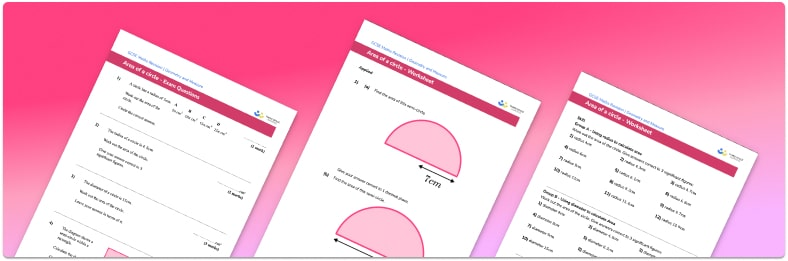# Area Of A Circle Worksheet• Section 1 of the printable area of a circle worksheet contains 20+ skills-based area of a circle questions, in 3 groups to support differentiation
• Section 2 contains 3 applied area of a circle questions with a mix of worded problems and deeper problem solving questions
• Section 3 contains 3 foundation and higher level GCSE exam style questions
• Answer keys and a mark scheme for all questions are provided
• Questions follow variation theory with plenty of opportunities for students to work independently at their own level
• All questions created by fully qualified expert secondary maths teachers
• Suitable for GCSE maths revision for AQA, OCR and Edexcel exam boards
• Printable worksheets are also available for related area of circles topics, including area of a parallelogram, area of compound shapes and circumference of the circle

• This field is for validation purposes and should be left unchanged.

You can unsubscribe at any time (each email we send will contain an easy way to unsubscribe). To find out more about how we use your data, see our privacy policy.

### Area of a circle at a glance

Area is the amount of space covered by a 2D shape and is measured in square units – metric units of area include  mm², cm² and m².

The formula for the area of a circle is πr², where r is the radius of the circle. If the radius is given, we need to square it and multiply it by π to find the area of the circle. If the diameter of a circle is given instead of its radius, we need to divide the diameter by 2 to find the radius, then proceed as above. If the area of a circle is given, students may be asked to work backwards to find the length of the radius. Here, we need to divide by π  and square root.

Students may sometimes be asked to leave their answer in terms of π; if this is not the case they will need to round the decimal answer to a given number of decimal places.

Application of this formula is fairly straightforward, but care must be taken as students can easily confuse this with the formula for the circumference of a circle.

This topic can be extended further to the area of a semicircle and other fractions of a circle. Word problems may require students to apply knowledge of compound shapes – for example, finding the area of a parallelogram with two circles cut from it, by finding the area of each circle and subtracting from the area of the parallelogram.

Looking forward, students can then progress to additional area worksheets and other geometry worksheets, for example an angles in polygons worksheet or area and circumference of a circle worksheet.For more teaching and learning support on Geometry our GCSE maths lessons provide step by step support for all GCSE maths concepts.

## Do you have KS4 students who need more focused attention to succeed at GCSE?There will be students in your class who require individual attention to help them succeed in their maths GCSEs. In a class of 30, it’s not always easy to provide.

Help your students feel confident with exam-style questions and the strategies they’ll need to answer them correctly with our dedicated GCSE maths revision programme.

Lessons are selected to provide support where each student needs it most, and specially-trained GCSE maths tutors adapt the pitch and pace of each lesson. This ensures a personalised revision programme that raises grades and boosts confidence.

Find out more